Note that these animations also show a simplified version of the behaviour of a vibrating bar like a xylophone key.

The first harmonic (also known as the fundamental) can be seen in Figure #1 below. The top plot with the red line is the graph typically seen in textbooks. It shows, in any frame, the instantaneous displacement of the air particles in the open pipe (shown as circles in the lower plot) relative to their "resting" position. When the red line is positive (higher), the air particles have moved to the right. When the red line is negative (lower) the air particles have moved to the left.

Notice that the red line (i.e. the xylophone bar) is free to move on each end (therefore it has two "low impedance terminations"). Similarly, the open ends of the pipe are low impedance. This means that the ends of the line can move, and so can the last air particles at the ends of the pipe (in fact, the particles at the end move in and out of the pipe - hence the requirement of a mouth correction or end correction in your calculations of the resonant frequency). This also means that there is a position in the centre of the pipe where the air particles do not move.

Notice as well that the resonance has a wavelength equal to two times the length of the pipe (just like a closed-closed pipe). This is more easily seen in the red-lined plot than the drawings of the particles in the pipe.

One important thing to note here is that, typically, in textbooks, you'll see a plot that shows either the pressure or the velocity of the air particles. The red lines shown here are neither of these. As I mentioned above, they're the displacement of the air particles, which is out of phase (but not opposite in polarity) with both the pressure and the velocity (which are 90 degrees out of phase with each other) at any point in the pipe.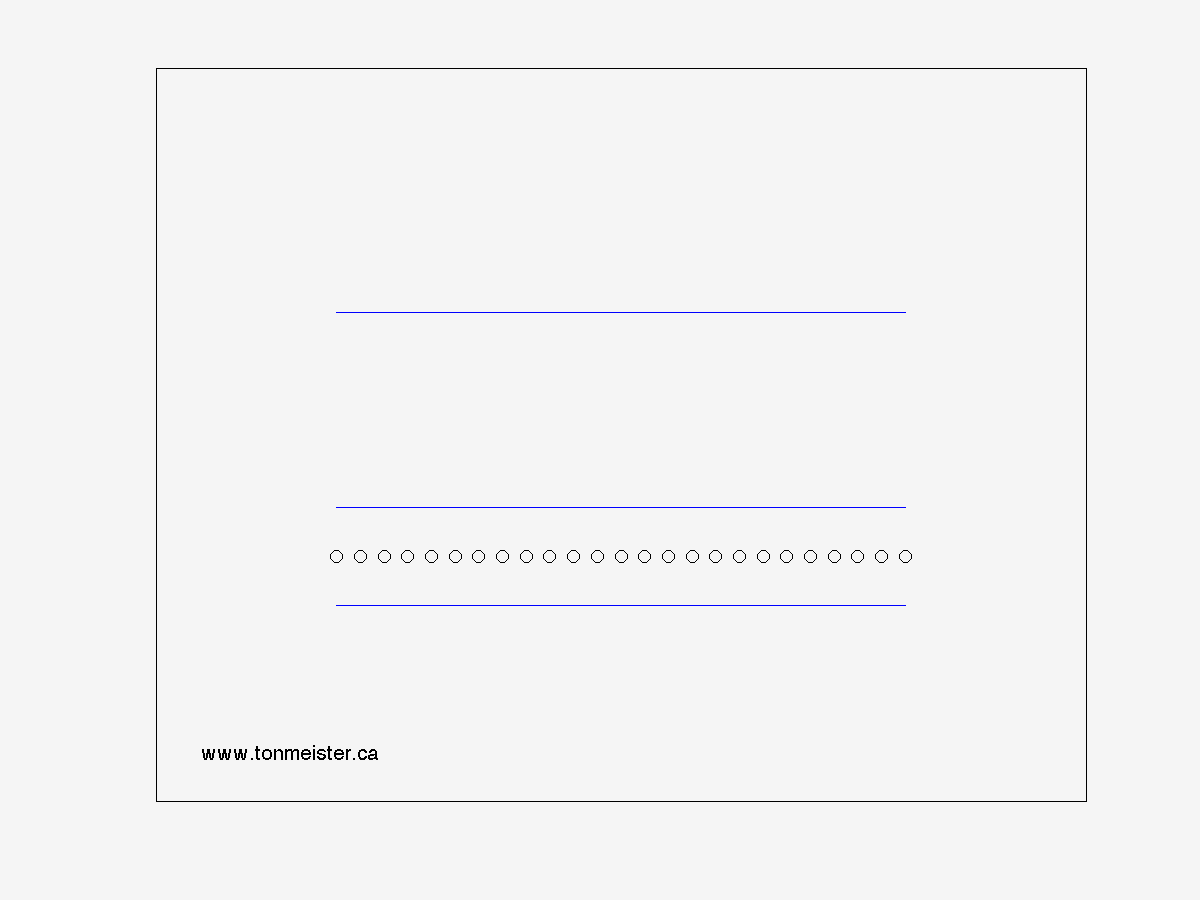Figure 1 - Harmonic #1

The second harmonic (shown below) occurs when the wavelength of the resonance equals the length of the pipe. This means that we have two points at the centre of the pipe where the air particle don't move. In the case of a xylophone bar, these two points are where the bar is sitting on the pads that hold it up. Therefore this is the lowest note that is allowed to vibrate on a xylophone key (since the first harmonic is damped by the supporting pads).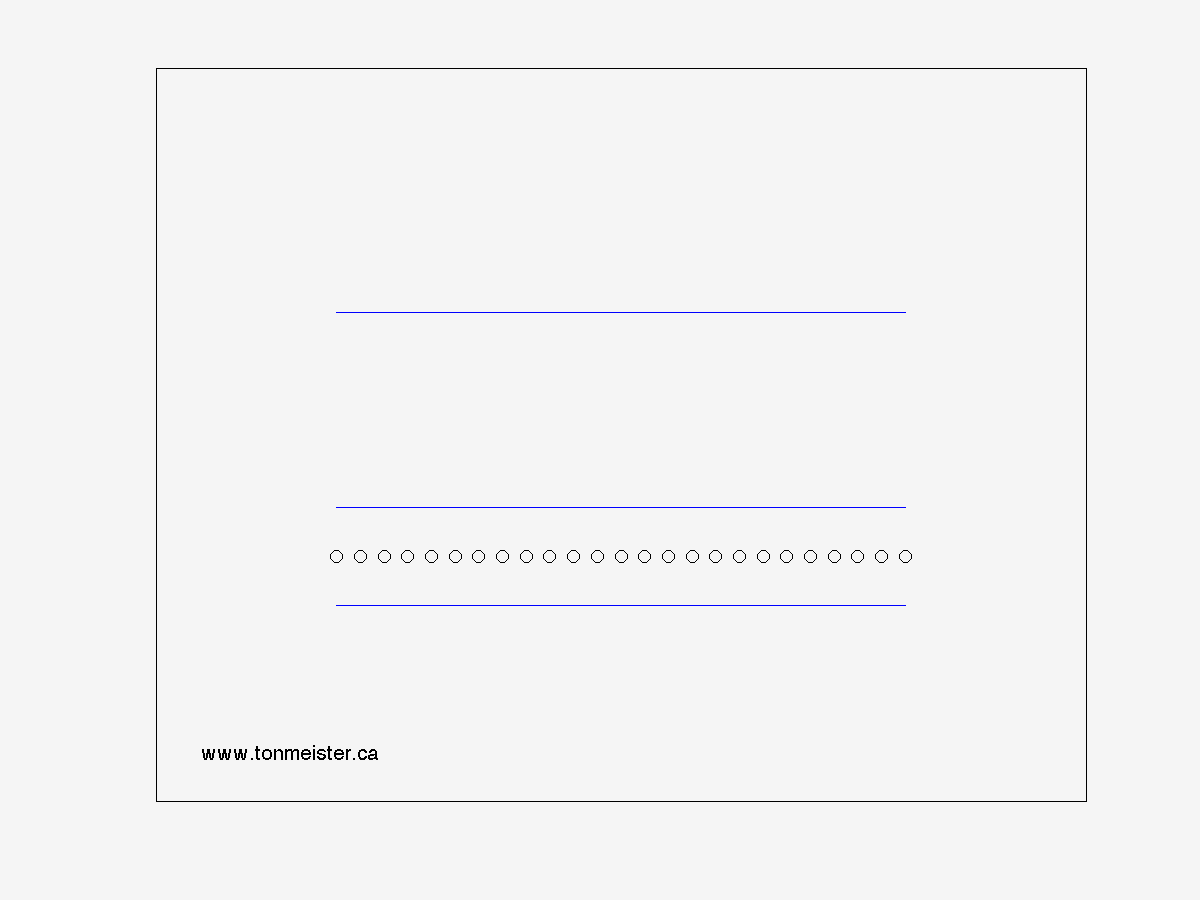Figure 2 - Harmonic #2

The third harmonic (shown below) occurs when the wavelength of the resonance equals 1.5 times the length of the pipe. This means that we have three points at the centre of the pipe where the air particles don't move.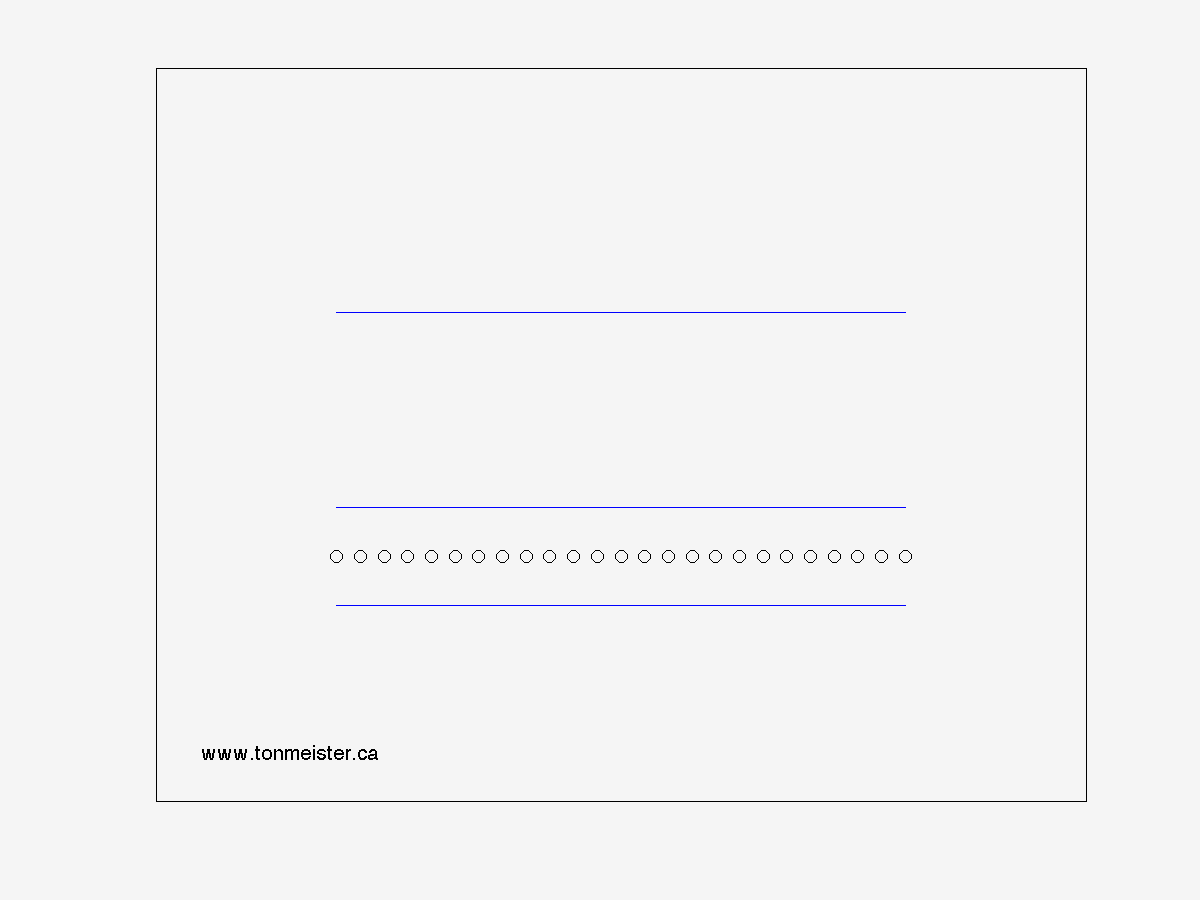Figure 3 - Harmonic #3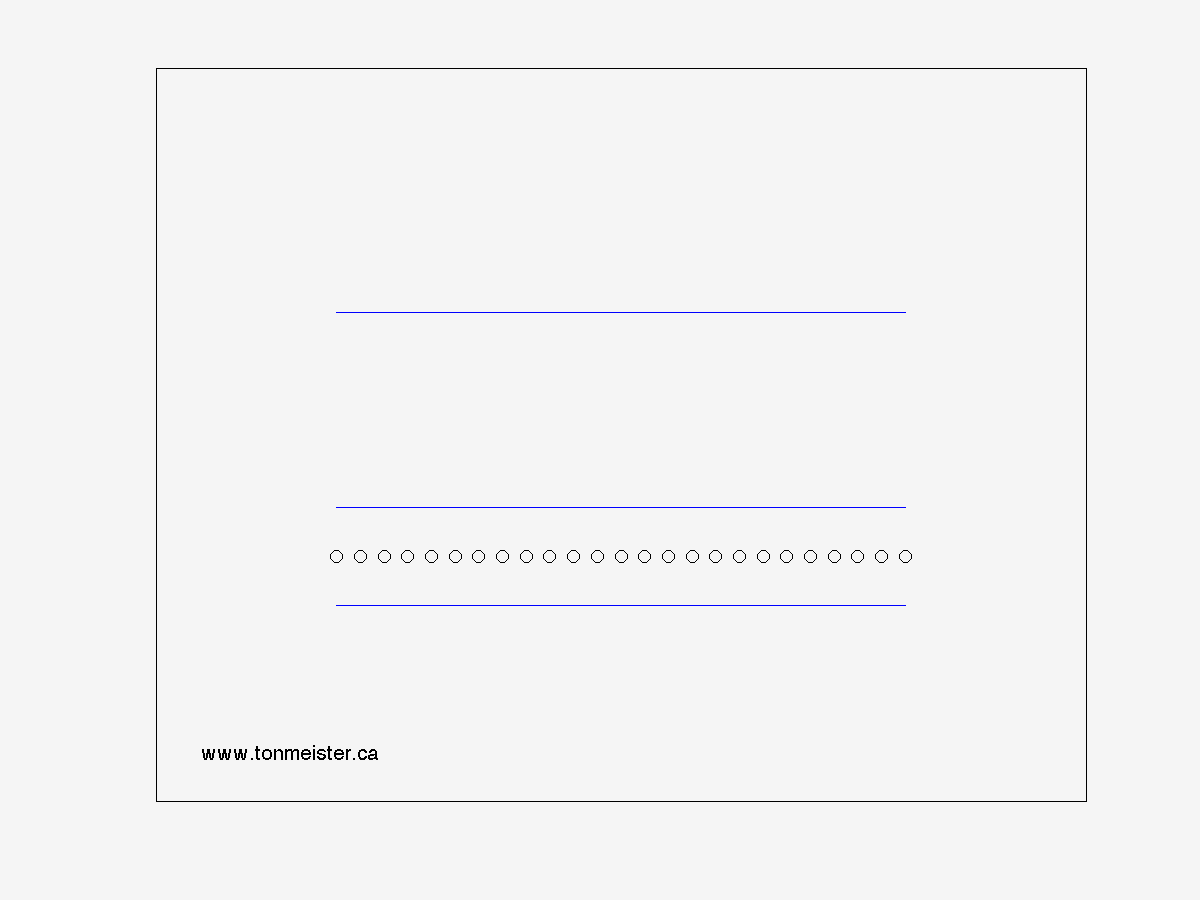Figure 4 - Harmonic #4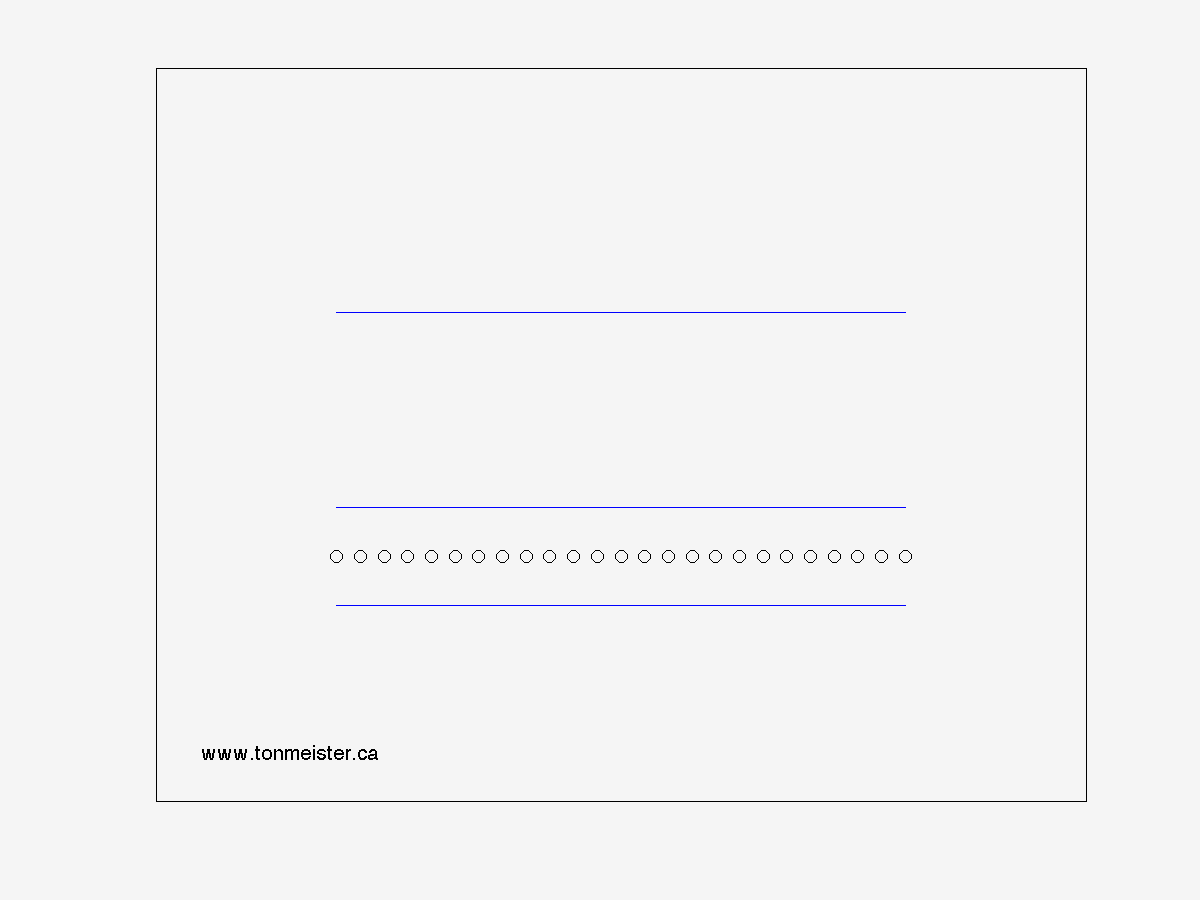Figure 5 - Harmonic #5